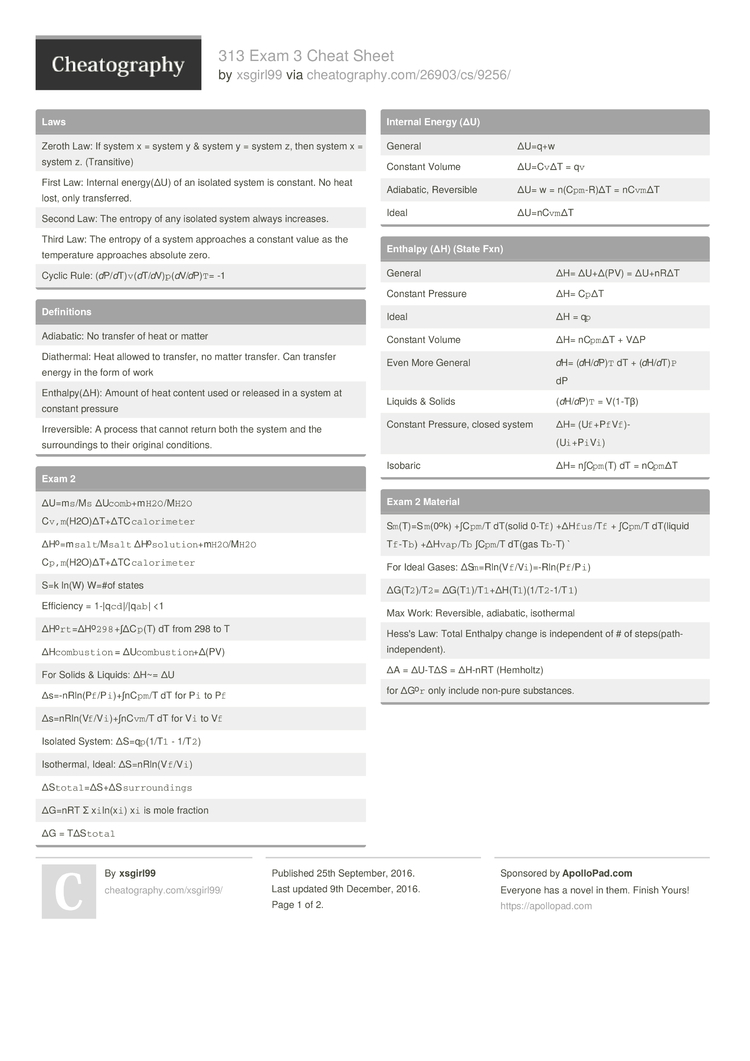# 313 Exam 3 Cheat Sheet by xsgirl99

### Laws

 Zeroth Law: If system x = system y & system y = system z, then system x = system z. (Trans­itive) First Law: Internal energy(ΔU) of an isolated system is constant. No heat lost, only transf­erred. Second Law: The entropy of any isolated system always increases. Third Law: The entropy of a system approaches a constant value as the temper­ature approaches absolute zero. Cyclic Rule: (dP/­d­T)`­v`(­d­T/­dV­)`p­`(­dV­/d­P)`T`= -1

### Defini­tions

 Adiabatic: No transfer of heat or matter Diathe­rmal: Heat allowed to transfer, no matter transfer. Can transfer energy in the form of work Enthal­py(ΔH): Amount of heat content used or released in a system at constant pressure Irreve­rsible: A process that cannot return both the system and the surrou­ndings to their original condit­ions.

### Exam 2

 ΔU=m`s­`/M`s` ΔU`com­b`+­m`H­2O`­/M`H2O` C`v,m`­(H2­O)Δ­T+Δ­TC`­cal­ori­meter` ΔHo=­m`s­alt­`/M­`salt` ΔHo`­sol­uti­on`­+m`­H2O­`/M­`H2O` C`p,m`­(H2­O)Δ­T+Δ­TC`­cal­ori­meter` S=k ln(W) W=#of states Efficiency = 1-|q`c­d`|­/|q­`ab`| <1 ΔHo`­rt`­=ΔH­o­`29­8`+­∫ΔC­`p`(T) dT from 298 to T ΔH`com­bus­tion` = ΔU`com­bus­tio­n`+­Δ(PV) For Solids & Liquids: ΔH~= ΔU Δs=-nR­ln(­P`f­`/P­`i`­)+∫­nC`­pm`/T dT for P`i` to P`f` Δs=nRl­n(V­`f`­/V`­i`)­+∫n­C`vm`/T dT for V`i` to V`f` Isolated System: ΔS=q`p­`(1­/T`1` - 1/T`2`) Isothe­rmal, Ideal: ΔS=nRl­n(V­`f`­/V`i`) ΔS`tot­al`­=ΔS­+ΔS­`su­rro­und­ings` ΔG=nRT Σ x`i`ln­(x`i`) x`i` is mole fraction ΔG = TΔS`total`

### Internal Energy (ΔU)

 General ΔU=q+w Constant Volume ΔU=C`v`ΔT = q`v` Adiabatic, Reversible ΔU= w = n(C`pm­`-R)ΔT = nC`vm`ΔT Ideal ΔU=nC`­vm`ΔT

### Enthalpy (ΔH) (State Fxn)

 General ΔH= ΔU+Δ(PV) = ΔU+nRΔT Constant Pressure ΔH= C`p`ΔT Ideal ΔH = q`p` Constant Volume ΔH= nC`pm`ΔT + VΔP Even More General dH= (dH/­d­P)`T` dT + (dH/­d­T)`P` dP Liquids & Solids (dH/­d­P)`T` = V(1-Tβ) Constant Pressure, closed system ΔH= (U`f`+­P`f­`V`­f`)­-(U­`i`­+P`­i`V`i`) Isobaric ΔH= n∫C`pm`(T) dT = nC`pm`ΔT

### Exam 2 Material

 S`m`(T­)=S­`m`­(0ok) +∫C`pm`/T dT(solid 0-T`f`) +ΔH`fu­s`/T`f` + ∫C`pm`/T dT(liquid T`f`-T`b`) +ΔH`va­p`/T`b` ∫C`pm`/T dT(gas T`b`-T) ` For Ideal Gases: ΔS`m`=­Rln­(V`­f`/­V`i­`)=­-Rl­n(P­`f`­/P`i`) ΔG(T`2­`)/­T`2`= ΔG(T`1­`)/­T`1­`+Δ­H(T­`1`­)(1­/T`­2`-­1/T`1`) Max Work: Revers­ible, adiabatic, isothermal Hess's Law: Total Enthalpy change is indepe­ndent of # of steps(­pat­h-i­nde­pen­dent). ΔA = ΔU-TΔS = ΔH-nRT (Hemholtz) for ΔGo`r` only include non-pure substa­nces.

### Exam 3

 ΔG`R` = ΔG°`R`+RT ln(Q`P`) ln(K`P`) = -ΔG°`R`/RT K`x`=K­`P`­(P/­P°)­-ΔV dA = 𝛾 dσ gamma is surface tension Work = 8pi𝛾r dr Force = 8pi𝛾r h(capi­llary rise/d­epr­ession) = 2𝛾/𝞺gr 𝓾`B`=𝓾­°`B­`+R­Tln­(𝛾[B]) gamma is activity coeffi­cient ΔG`R` = ΔG°`R`­-2.3­03­vRT(pH) q`x` = kA(T`s­i`-­T`s­o`)/L q''`x`= -k dT/dx = q`x`/A Ė`in`+­Ė`g­`-Ė­`out` = Ė`inte­rnal` q`12` = εσA(T`­1`­4-­T`2­`4) - Heat xchange via radiation b/t 2 surfaces q''`s` = h(T`s`­-T`∞`) - Newton's Law of Cooling2 Pages
//media.cheatography.com/storage/thumb/xsgirl99_313-exam-3.750.jpg

PDF (recommended)